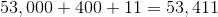ISEE Lower Level Quantitative : How to add

Example Questions

Example Question #11 : How To Add

Which of the following is the sum of two-thirds and five-sixths?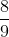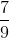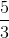Explanation: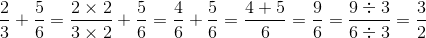Example Question #12 : How To Add

Which of the following is the sum of one-tenth and one-sixth?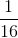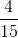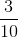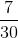Explanation: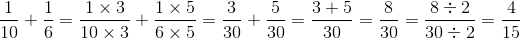Example Question #414 : Isee Lower Level (Grades 5 6) Quantitative Reasoning

The time is 8:45 AM. What time will it be in two hours and thirty minutes?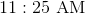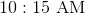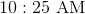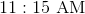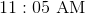Explanation:

You can add two hours and thirty minutes to 8:45 AM as follows: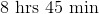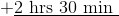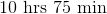Since one hour is equal to 60 minutes, divide 75 minutes by 60; this is 1 hour 15 minutes, so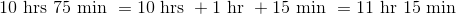making the time 11:15 AM.

Example Question #411 : Operations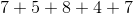The correct response is not given among the other responses.Explanation:

The sum of two or more numbers in modulo 9 arithmetic is the remainder of the sum when it is divided by 9. Since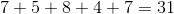and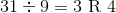,

then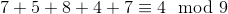The correct response is 9.

Example Question #416 : Isee Lower Level (Grades 5 6) Quantitative Reasoning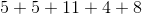Explanation:

The sum of two or more numbers in modulo 12 arithmetic is the remainder of the sum when it is divided by 12. Since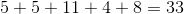and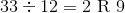,

then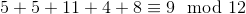The correct response is 9.

Example Question #417 : Isee Lower Level (Grades 5 6) Quantitative Reasoning

Give the sum of 0.4, 0.44, and 0.444.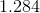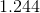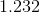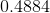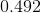Explanation:

Write the two numbers vertically with decimal points aligned, adding two zeroes to the 0.4 and one to the 0.44. Then add vertically: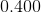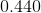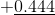Example Question #411 : Isee Lower Level (Grades 5 6) Quantitative Reasoning

Which expression is equal to three times J plus four?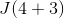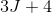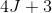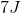Explanation:

Three times J is equal to 3J. Therefore, three times J plus four is equal to 3J + 4.

Thus, the correct answer is:Example Question #411 : Isee Lower Level (Grades 5 6) Quantitative Reasoning

Suzie had twice as many marbles as Sandy. Each child was given a bag of 60 marbles, and now Suzie has 110 marbles. How many marbles does Sandy have now?Explanation:

Suzie has 110 marbles; she had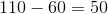marbles before being given the bag of 60. Sandy had half this, or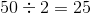marbles; after being given her bag of 60, she now has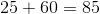marbles.

Example Question #420 : Isee Lower Level (Grades 5 6) Quantitative Reasoning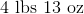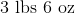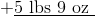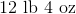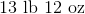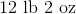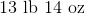Explanation:

First, add pounds and ounces separately.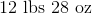Since 16 ounces make one pound, we divide 28 by 16: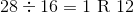28 ounces is equal to 1 pound 12 ounces, so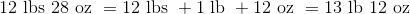Example Question #11 : How To Add

What number is equal to fifty-three thousand, four hundred and eleven?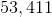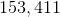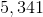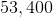Fifty-three thousand equals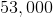, four hundred equals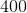, and eleven equals